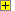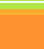Zener diode as a
voltage regulatorCalculation of values for a zener diode.
The power supply shown below uses a   V rated zener diode to give a regulated output of   V from an input voltage of   V
The zener diode must conduct at least   mA
The maximum power supply output current required is   mA

Zener diode as
a voltage regulator

Vin =   V
Vout =   V
minimum current through zener diode (D1) =   mA
maximum power supply output current =   mA

Calculate: (answers to 1dp or integers as appropriate)
 * the maximum current throught resistor (R1) = mA * the voltage drop across R1 = V * the value of R1 under these conditions = Ω
The current drawn from the power supply falls to zero.

Calculate: (answers to 1dp or integers as appropriate)
 * the current now flowing through the zener diode = mA * the power now dissipated by the zener diode = mWShow Calculator

home